# 机器学习A-Z～Logistic Regression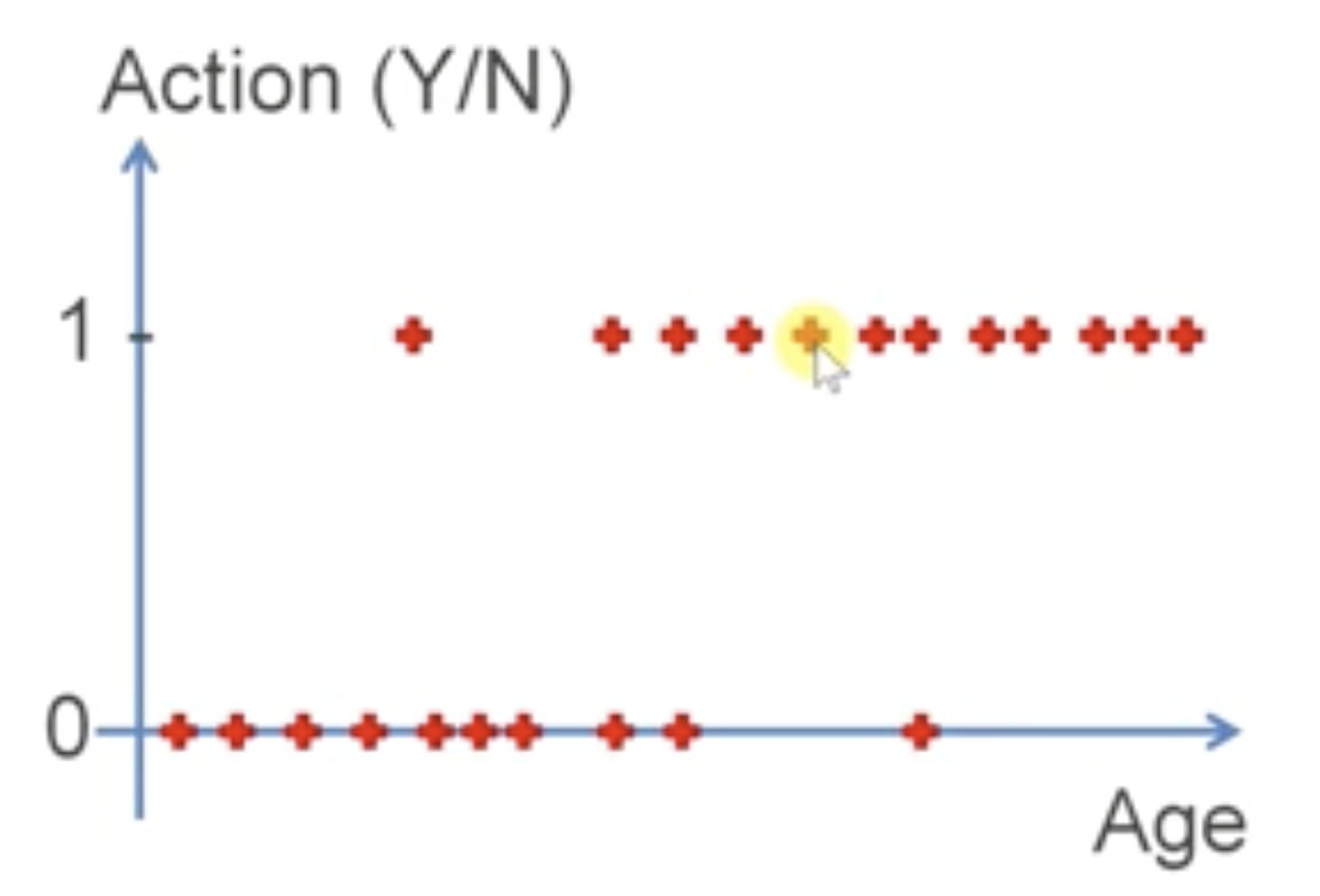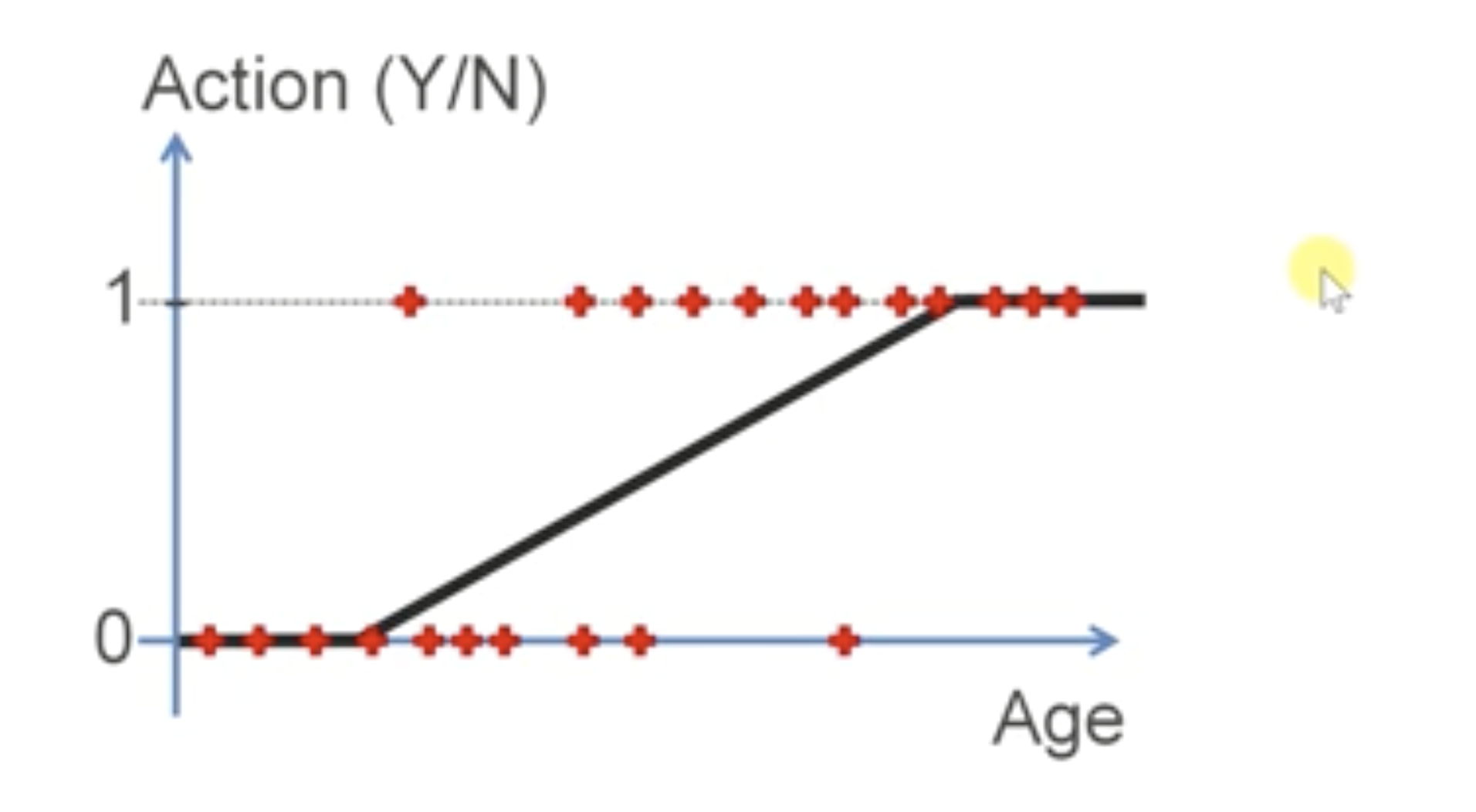p = \frac{1}{1+e^{-y}}

ln(\frac{p}{1-p}) = b_0 + b_1 * x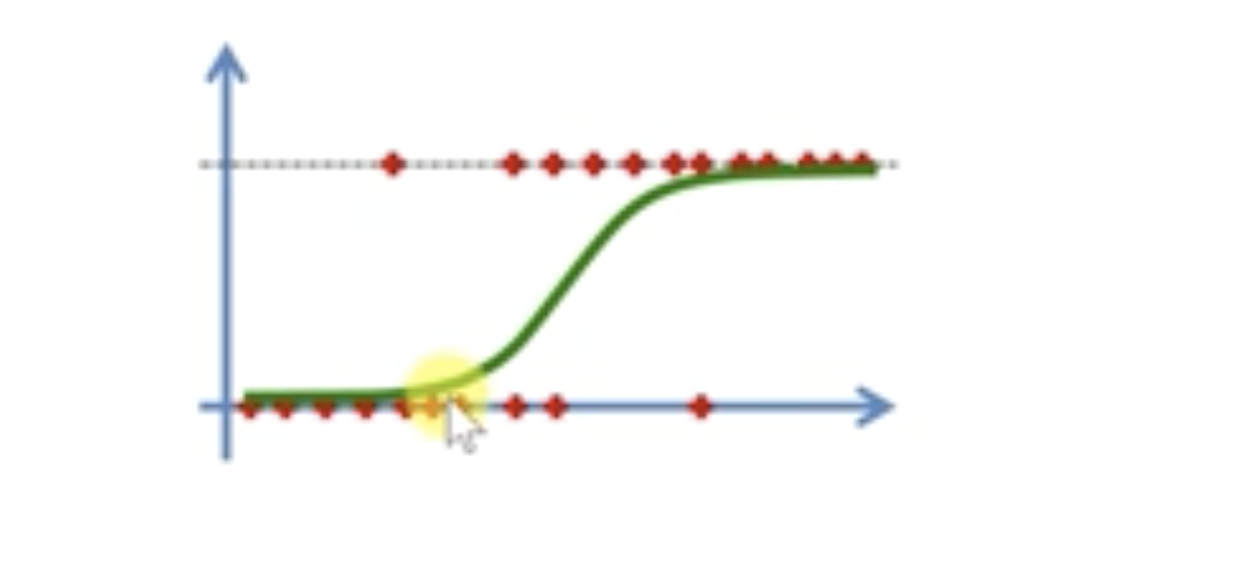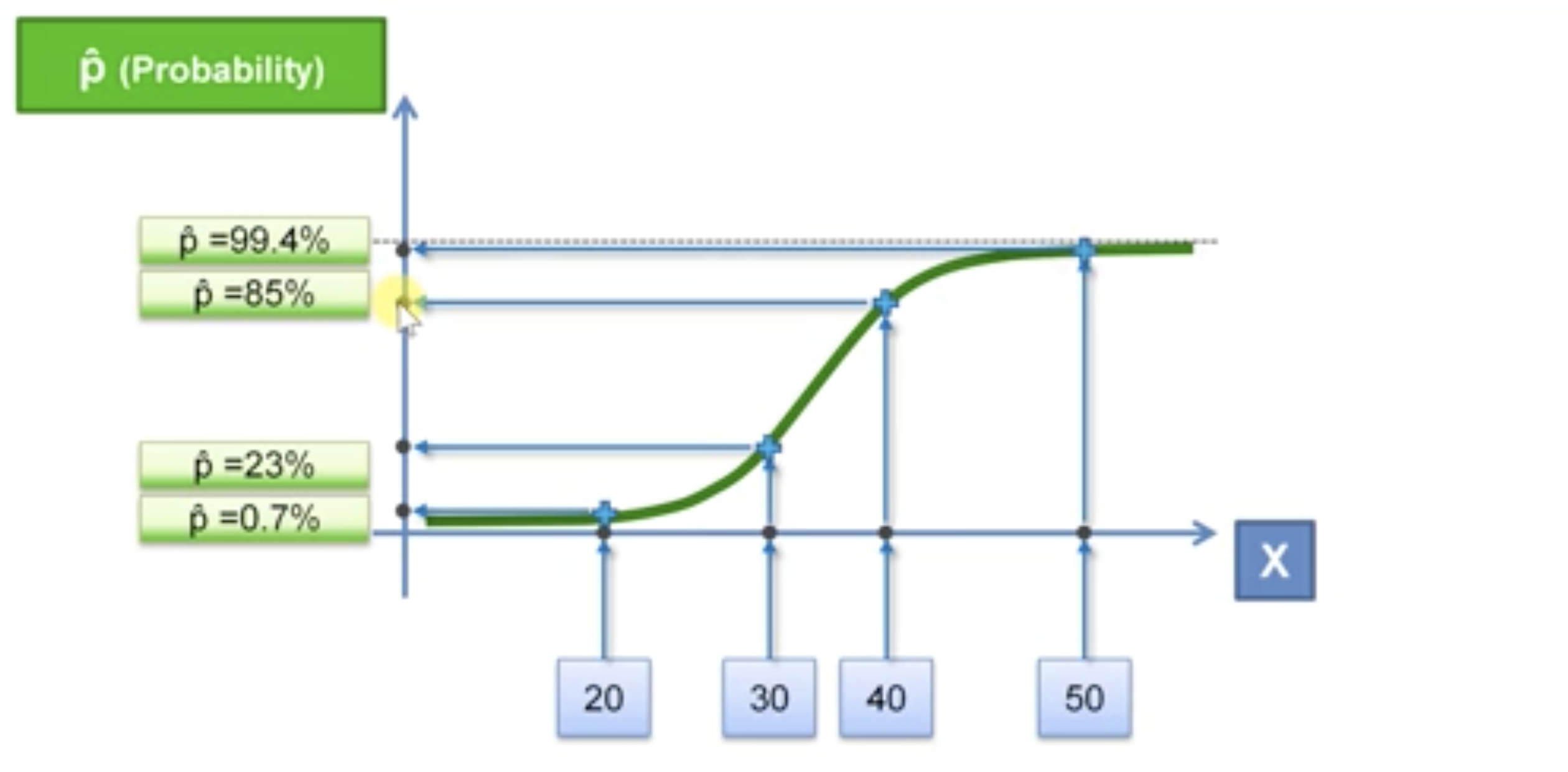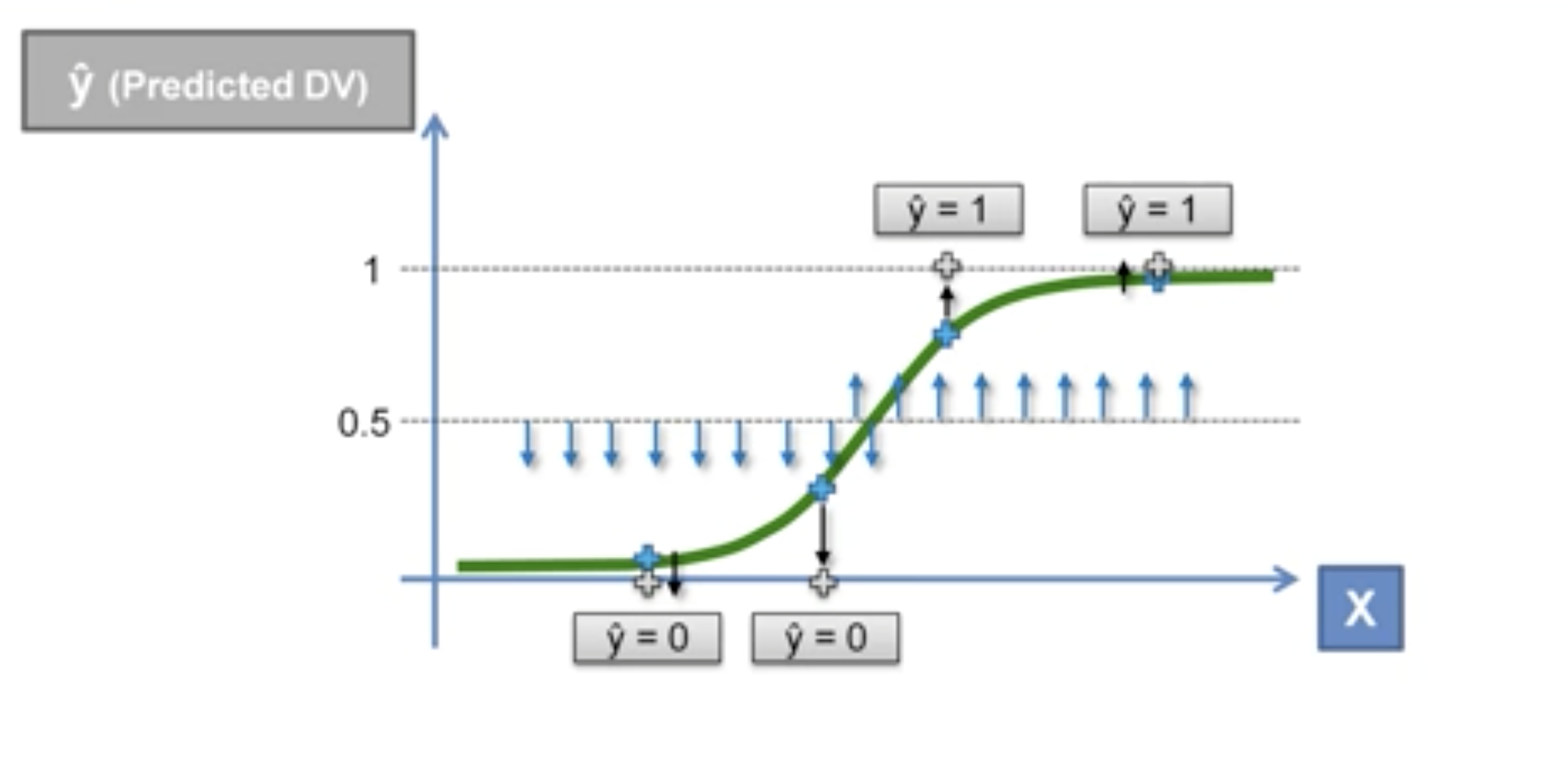User ID Gender Age EstimatedSalary Purchased
15624510 Male 19 19000 0
15810944 Male 35 20000 0
15668575 Female 26 43000 0

import pandas as pd
from sklearn.model_selection import train_test_split
from sklearn.preprocessing import StandardScaler
from sklearn.linear_model import LogisticRegression
from sklearn.metrics import confusion_matrix

X = dataset.iloc[:, [2, 3]].values
y = dataset.iloc[:, 4].values

X_train, X_test, y_train, y_test = train_test_split(X, y, test_size=0.25, random_state=0)

# feature scaling
sc_X = StandardScaler()
X_train = sc_X.fit_transform(X_train)
X_test = sc_X.transform(X_test)

# Fitting Logistic Regression to the Training set
classifier = LogisticRegression(random_state=0)
classifier.fit(X_train, y_train)

# Predicting the Test set results
y_pred = classifier.predict(X_test)

# Making the Confusion Matrix(混淆矩阵)
cm = confusion_matrix(y_test, y_pred)


array([[65,  3],
[ 8, 24]])


X_set, y_set = X_train, y_train
X1, X2 = np.meshgrid(np.arange(start=X_set[:, 0].min() - 1, stop=X_set[:, 0].max() + 1, step=0.01),
np.arange(start=X_set[:, 1].min() - 1, stop=X_set[:, 1].max() + 1, step=0.01))
plt.contourf(X1, X2, classifier.predict(np.array([X1.ravel(), X2.ravel()]).T).reshape(X1.shape),
alpha=0.75, cmap=ListedColormap(('red', 'green')))
plt.xlim(X1.min(), X1.max())
plt.ylim(X2.min(), X2.max())
for i, j in enumerate(np.unique(y_set)):
plt.scatter(X_set[y_set == j, 0], X_set[y_set == j, 1],
c=ListedColormap(('orange', 'blue'))(i), label=j)
plt.title('Classifier (Training set)')
plt.xlabel('Age')
plt.ylabel('Estimated Salary')
plt.legend()
plt.show()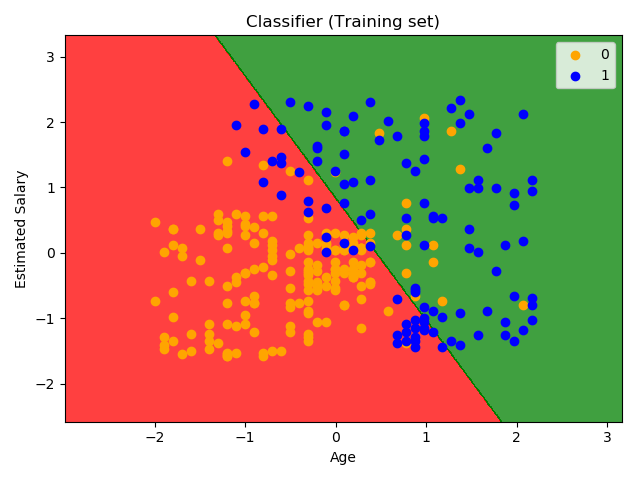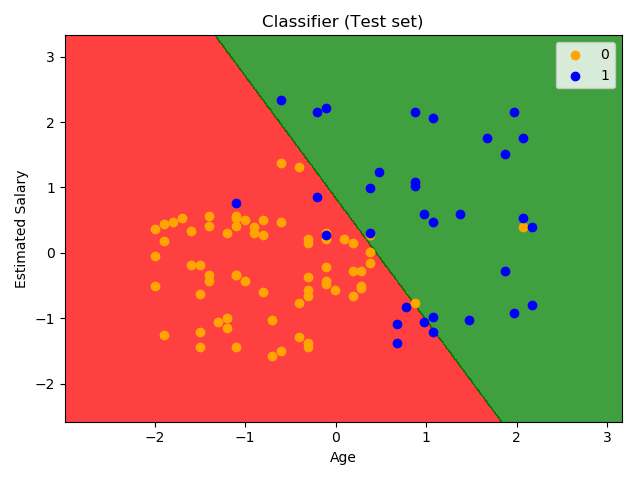classifier = LogisticRegression(random_state=0)
classifier.fit(X_train, y_train)

# Predicting the Test set results
y_pred = classifier.predict(X_test)